# 二、为什么我们需要并发？

（https://www.karlrupp.net/2015/06/40-years-of-microprocessor-trend-data/）

# 三、单线程中的并行执行

## 3.3 TMAM

TMAM（Top-down Microarchitecture Analysis Method）是一种利用CPU内部PMU（Performance Monitoring Unit）计数器来从上至下分解定位部件瓶颈的手段。例如在最顶层，首先以标定最大指令完成速率为标准（例如Skylake上为单周期4条微指令），如果无法达到标定，则认为瓶颈在于未能充分利用部件。进一步细分以指令池为分界线，如果指令池未满，但是取指部件又无法满负荷输出微指令，就表明『前端』存在瓶颈。另一种无法达到最大指令速率的因素，是『前端』虽然在发射指令到指令池，但是因为错误的预测，最终没有产出有效结果，这类损耗则被归入『错误预测』。除此以外的问题就是因为指令池调度执行能力不足产生的反压停顿，这一类被归为『后端』瓶颈。进一步例如『后端』瓶颈还可以根 据，停顿发生时，是否伴随了ALU利用不充分，是否伴随了Load/Store Buffer满负荷等因素，继续进行分解细化，形成了一套整体的分析方法。例如针对Intel，这一过程可以通过pmu-tools来被自动完成，对于指导精细化的程序瓶颈分析和优化往往有很大帮助。

``````int array;
for (size_t i = 0; i < 1024; i += 2) {
int a = array[i];
int b = array[i + 1];
for (size_t j = 0; j < 1024; ++j) {
a = a + b;
b = a + b;}
array[i] = a;
array[i + 1] = b;
}
``````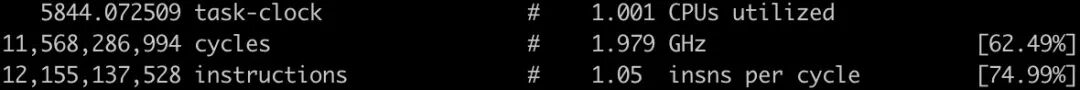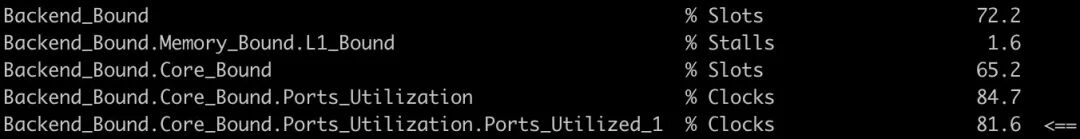`int array;``for (size_t i = 0; i < 1024; i += 4) {` `int a = array[i];` `int b = array[i + 1];` `int c = array[i + 2];` `int d = array[i + 3];` `for (size_t j = 0; j < 1024; ++j) {` `a = a + b;` `b = a + b;` `c = c + d;` `d = c + d;` `}` `array[i] = a;` `array[i + 1] = b;` `array[i + 2] = c;` `array[i + 3] = d;``}`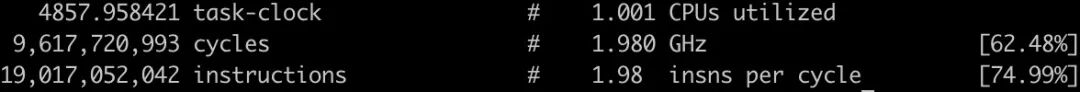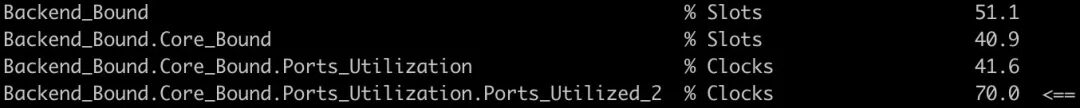``````struct Line {
char data;
};
Line* lines; // 其中乱序存放多个缓存行
for (size_t i = 0; i < 1024; ++i) {
Line* line = lines[i];
for (size_t j = 0; j < 64; ++j) {
line->data[j] += j;
}
}
``````

``````for (size_t i = 0; i < 1024; i += 2) {
Line* line1 = lines[i];
Line* line2 = lines[i + 1];
...
for (size_t j = 0; j < 64; ++j) {
line1->data[j] += j;
line2->data[j] += j;
...
}
}
``````

# 四、多线程并发中的临界区保护

## 4.1 什么是临界区

### **4.2 **无锁不是万能的

``````// 在一个cache line上进行指定步长的斐波那契计算来模拟临界区计算负载
uint64_t calc(uint64_t* sequence, size_t size) {
size_t i;
for (i = 0; i < size; ++i) {
sequence[(i + 1) & 7] += sequence[i & 7];
}
return sequence[i & 7];
}
{   // Mutual Exclusion
::std::lock_guard<::std::mutex> lock(mutex);
}
{   // Lock Free / Atomic CAS
auto next = current;
do {
next = current + calc(sequence, workload);
} while (!atomic_sum.compare_exchange_weak(
current, next, ::std::memory_order_relaxed));
}
{   // Wait Free / Atomic Modify
}
``````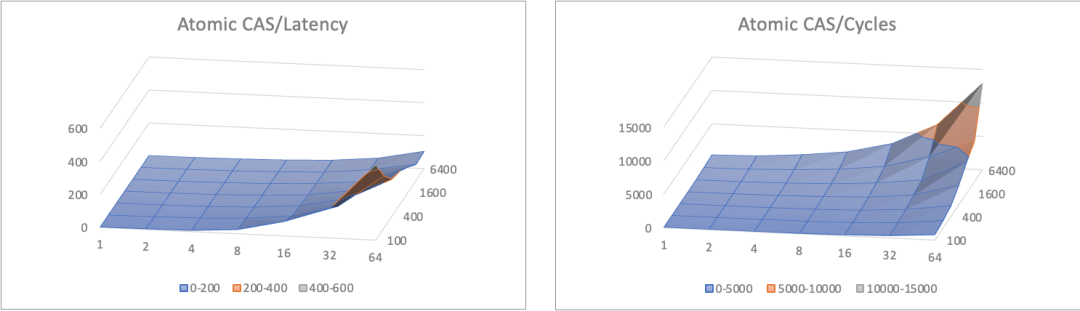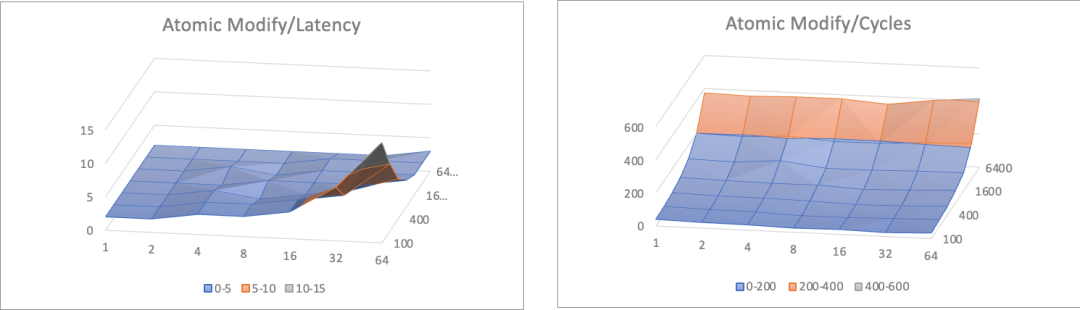1、基于FAA的Wait Free模式各方面都显著胜过其他方法；

2、无锁算法相比互斥算法在平均吞吐上有一定优势，但是并没有达到数量级水平；

3、无锁算法随竞争提升（临界区大小增大，或者线程增多），cpu消耗显著上升；

## 4.3 并发计数器优化案例

 local count get value bvar::IntRecorder 16 7 babylon::IntRecorder 1377 14 bvar::Percentile 938 28 babylon::Percentile 66 14

## **4.4 **并发队列优化案例

 latency boost::lockfree::queue 35075 tbb::concurrent_queue 4614